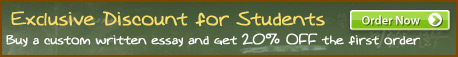### The importance of arithmetic in math

Title: The importance of arithmetic in math
Category: /Science & Technology/Mathematics
Details: Words: 754 | Pages: 3 (approximately 235 words/page)
The importance of arithmetic in math
Arithmetic is the ABC of math. Addition, subtraction, multiplication, and division are the basics of math and every math operation known to humankind. In one way or another, every equation, graph, and an enormous amount of other things can be broken down into the ABC's of math, the four basic operations. As people say, math is a language, and addition subtraction, multiplication, and division are its alphabet, along with the number line as well. The …showed first 75 words of 754 total…
You are viewing only a small portion of the paper.